Question

While describing a circular orbit 300 mi above the earth a space vehicle launches a 6000-lb communications satellite. Determine the additional energy required to place the satellite in a geosynchronous orbit at an altitude of 22,000 mi

1.mocmien2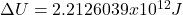Explanation:

While the satellite is in the space vehicle, it has the next potential energy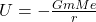where G is the gravitational constant

m is the satellite’s mass in kilograms

Me is the earth’s mass

r is the orbit’s radius from to the earth’s center in meters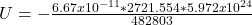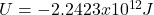The additional energy required is the difference between this energy and the energy that the satellite would have in an orbit with an altitude of 22000 mi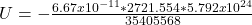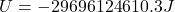Then